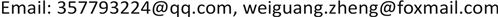OJAVOpen Journal of Acoustics and Vibration2328-0530Scientific Research Publishing10.12677/OJAV.2018.62005OJAV-25267OJAV20180200000_86102745.pdf数学与物理 基于Virtual.Lab Acoustics的管道壁面声阻抗优化分析 Optimization and Analysis of Acoustic Impedance of Duct Wall Based on Virtual.Lab Acoustics 伟光2121泽宇21荣江21桂林电子科技大学，机电工程学院，广西 桂林null2805201806023645© Copyright 2014 by authors and Scientific Research Publishing Inc. 2014This work is licensed under the Creative Commons Attribution International License (CC BY). http://creativecommons.org/licenses/by/4.0/Copyright © 2018 by authors and Hans Publishers Inc.1. 引言

2. 有限元管道声模型

( i k + M ∂ ∂ x ) 2 p − ∇ 2 p = 0 (1)

∂ p ∂ y = 0 , ∂ p ∂ z = 0 (2)

∂ p ∂ y = − i k ( 1 + M i k ∂ ∂ x ) 2 p Z (3)

p = ∑ i = 1 q N i e p i = [ N ] T [ p i ] (4)

∫ V p ˜ [ ( i k + M ∂ ∂ x ) 2 p − ∇ 2 p ] d V = ∫ Ω p ˜ ( ∂ p ∂ n ) d Ω (6)

∂ p ∂ n = 0 (7)

∂ p ∂ n = − j ρ 0 ω u n (8)

∂ p ∂ n = − j ρ 0 ω p Z n (9)

Ω = Ω r + Ω v + Ω z (10)

∫ { [ K ] + j ρ 0 ω 1 Z n [ C ] − k 0 2 [ C ] } [ p i ] = − j ρ 0 ω u n (11)

[ K ] = ∫ r [ N g ] ⋅ [ N g ] T d V [ M ] = ∫ r [ N ] ⋅ [ N ] T d V

[ C ] = ∫ Ω Z [ N ] ⋅ [ N ] T d V

[ F ] = ∫ Ω v [ N ] d Ω

3. 壁面声衬最优声阻抗

3.1. 声学仿真优化计算

Optimal acoustic impedance and transmission loss at specific frequency (M = 0

5000.059 − 0.120i0.06 − 0.12i40.739.8
7000.089 − 0.168i0.09 − 0.17i44.341.9
9000.113 − 0.225i0.11 − 0.23i41.840
11000.140 − 0.280i0.14 − 0.28i50.949.2
13000.165 − 0.337i0.16 − 0.33i39.939.2
15000.190 − 0.412i0.19 − 0.42i58.859.1

Optimal acoustic impedance and transmission loss at specific frequency (M = 0.1

5000.030 − 0.036i0.03 − 0.035i29.431.1
7000.051 − 0.067i0.05 − 0.065i45.142.5
9000.079 − 0.117i0.08 − 0.12i50.148.8
11000.096 − 0.171i0.09 − 0.17i34.935.6
13000.132 − 0.232i0.12 − 0.23i48.249
15000.152 − 0.320i0.15 − 0.31i41.342.7

3.2. 最优阻抗值

3.3. 不同边界条件下最优阻抗值

4. 声阻抗参数灵敏度分析

5. 结论

1) 无论管道是否存在切向气流时，最优阻抗值的实部都与频率成正比，虚部与频率成反比且一直为负值。

2) 当管道内存在均匀流动时，最优阻抗值有明显的变化。实部相比于无流情况下有所减小，虚部则增大。

3) 声衬长度对最优阻抗值的虚部影响不大；声衬铺设方式的不同对阻抗最优值有较明显的影响。

4) 声阻抗值的较小变化都会令管道的传递损失发生显著的改变。